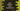# Different Dart class constructors# Introduction :

You must be familiar with the term class if you have worked on any object-oriented programming language. Objects are an instance of classes. Class is a blueprint and objects are created using that blueprint.

A constructor is a method defined in a class that is called while initializing an object for that class. It can have parameters to take during the initialization. Those arguments are assigned to the newly created object.

Interestingly, Dart provides a couple of different ways to write constructors. In this tutorial, I will show you the different ways that we have for initialization.

## Different types of constructors in dart :

### Default constructor :

Each class has a constructor by default. It doesn’t take any parameter and simply creates one object for that class. For example :

``````class Student{
String name;
int age;
}

main() {
var s = new Student();
print(s.name);
print(s.age);
}``````

Here, we are creating one instance s for the class Student. new keyword is optional. We are not initializing the variables name and age. So, it will print null for both.

### Generative constructor :

Using a generative constructor, we can create one new instance and assign values to the variables.

``````class Student{
String name;
int age;

Student(String name, int age){
this.name = name;
this.age = age;
}
}

main() {
var s = Student("Alex", 20);
print(s.name);
print(s.age);
}``````

this keyword is used to refer to the current instance. If the arguments and variables have different names, no need to use it.

This is a common way to create an object using a constructor in most programming languages. Dart provides an easier way to do that :

``````class Student{
String name;
int age;

Student(this.name, this.age);
}

main() {
var s = Student("Alex", 20);
print(s.name);
print(s.age);
}``````

### Named constructors :

Named constructors are useful if you have multiple ways to initialize a class. For example :

``````class Student{
String name;
int age;

Student.withName(this.name);
Student.withAge(this.age);
}

main() {
var s = Student.withName("Alex");
print(s.name);
print(s.age);
}``````

We have two different named constructors here. Named constructors are useful to get more clarity on initialization.

### Initialize variables before body :

We can initialize the instance variables before the constructor body runs :

``````class Student{
String name;
int age;

Student.withName(String studentName) : name = studentName{
print("Initialized a student with name \$name");
}
}

main() {
var s = Student.withName("Alex");
}``````

It will assign the variable name before executing the print command.

### Redirect to a different constructor :

Redirecting a constructor means calling one different constructor from another constructor.

``````class Student{
String name;
int age;

Student(this.name,this.age);

Student.withName(String name) : this(name,-1);
}

main() {
var s = Student.withName("Alex");
print("Name = \${s.name} and age = \${s.age}");
}``````

Here, we have one named constructor withName that takes one string name. It calls another constructor, that is written after the colon to assign both name and age as -1 to the current object.

If you execute the program, it will print :

``Name = Alex and age = -1``

### Constant constructors :

constant constuctors are used to create objects that never changes. Keyword const is used before the constructor name to create constant constructors. Also, the variables should be final :

``````class Student{
final String name;
final int age;

const Student(this.name,this.age);
}

main() {
var s = const Student("Alex", 10);
print("Name = \${s.name} and age = \${s.age}");
}``````

We are using const before the object initialization. If you use const, it will always create one object of the same instance. If you don’t use it, it will create one object with a different instance.

### Factory constructors :

Factory constructor doesn’t create any new instance of the class always. It checks the cache and it might return an instance from the cache or it might return an instance of a subtype. It doesn’t have access to this and we can invoke it like any other constructors. factory keyword is used for factory constructors.

``````class Student{
String name;
int age;

Student(this.name,this.age);

factory Student.create(String studentName,int studentAge){
return Student(studentName,studentAge);
}
}

main() {
var s = Student.create("Alex", 10);
print("Name = \${s.name} and age = \${s.age}");
}``````